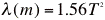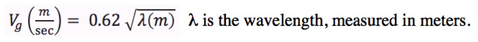|

# Question of the Day: Ocean Waves #2

## Question:

Surfers know that the best waves from distant storms have periods of about 14 seconds. The object of this activity is to compute how long it takes the 14 second period waves to travel 6,000 km across the pacific.

Facts:

The formula for travel time is:

time (secs) =distance (km)/speed (km/sec)

A wave is a deep water wave if the depth > wavelength/2

A wave is a shallow water wave if depth < wavelength/20

To figure out whether it's a deep or shallow water wave, you need to find its wavelength.

a) The formula for wavelength vs. period isT, the period, is in seconds. Make this calculation to find the wavelength of the wave.

b) Given what you know about the Pacific Ocean depth, do you expect this wave to be a deep water, or a shallow water wave?

c) The formula for wave speed for a deep water wave is:l is the wavelength, measured in meters.

Calculate the speed in km/sec, for the 14 sec period wave.

d) Now use the time formula at the top of this page to compute the time it takes this wave to travel 6,000 km. Enter your answer below.

## References and Notes:

Question of the Day is a method used by Dr. William Prothero in his Oceanography Course at the University of California, Santa Barbara.

The Question of the Day page with more examples and tips on using them is part of the Starting Point: Interactive Lectures Module.

## Subject

Geoscience:Oceanography:Physical

## Resource Type

Activities:Classroom Activity:Short Activity

## Special Interest

Quantitative

College Lower (13-14):Introductory Level

## Quantitative Skills

Problem Solving:Equations, Arithmetic/Computation, Algebra